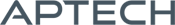# Nonlinear Equations MT

Nonlinear Equations MT

Availability: Out of stock

The Nonlinear Equations MT applications module (NLSYS) solves systems of nonlinear equations where there are as many equations as unknowns.
Overview

## Nonlinear Equations MT

The Nonlinear Equations MT applications module (NLSYS) solves systems of nonlinear equations where there are as many equations as unknowns. This application is thread-safe and takes advantage of structures found in later versions of GAUSS.

The functions must be continuous and differentiable. You may provide a function for calculating the Jacobian, if desired. Otherwise NLSYS will compute the Jacobian numerically. NLSYS solves a system of nonlinear equations using a quasi-Newton algorithm with Broyden's secant updated method. You an choose either a line search algorithm or a hookstep method.

Platform: Windows, Mac and Linux.

Requirements: GAUSS/GAUSS Engine/GAUSS Light v8.0 or higher.

Product Inquiry

2 + 6 = enter the result ex:(3 + 2 = 5)

﻿